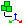# Transformations

Transformation entities define solver transformations, and are used to define a transformation sequence in a Position entity, to be applied on a set of nodes or on a SolverSubmodel.## Abaqus

Transformation entities hold the transformation information that is provided in the datalines of the *NMAP keyword.
Card Description
*NMAP Maps nodes from one coordinate system to another, and rotates, translates, or scales the nodal coordinates.
Note: More than one transformation can be applied on a given node set using additional *NMAP keywords.

A given transformation can be applied multiple times using separate *NMAP keywords for each required instance.

The coordinates for Point A and Point B cannot be the same.

If the Magnitude is greater than 0, the nodes will be translated in the direction of the vector AB; if the Magnitude is less than 0, the nodes will be translated in the opposite direction of the vector AB. If the Magnitude is equal to 0, an error message will be issued when the transformation is applied.

A positive angle results in a counter clockwise rotation; a negative angle results in a clockwise rotation. If the Angle is equal to 0, an error message will be issued when the transformation is applied.

If the scale factor along the X, Y, and Z axes are uniform, then the Uniform Scale checkbox can be selected and one Scale size can be specified. If the scale factor is different along each axis, then it can be entered separately by clearing the Uniform scale checkbox.

Transformations entities hold the Radioss keywords /TRANSFORM.
Supported Card Solver Description
//SUBMODEL Defines a part of the model with separate definition of numeration, unit system and Radioss version.
/TRANSFORM/TRA Defines a translation for a set of nodes or a submodel.

A transformation cannot be applied on a node set and a submodel using the same keyword.

/TRANFORM/ROT Defines a rotation for a set of nodes or a submodel.

A transformation cannot be applied on a node set and a submodel using the same keyword.

/TRANFORM/SCA Defines scaling factors in X,Y,Z directions for a set of nodes.
/TRANSFORM/SYM Defines a symmetry on a set of nodes, normal to the plane defined by a vector.